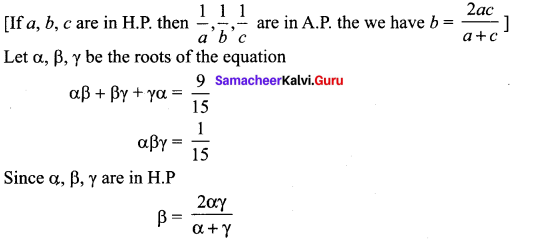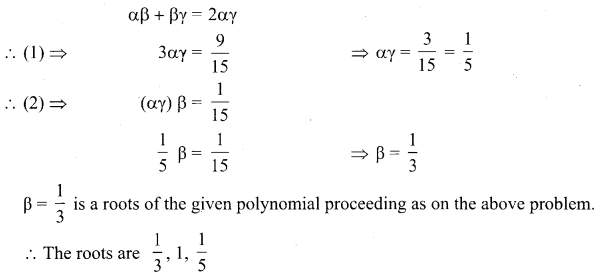## Tamilnadu Samacheer Kalvi 12th Maths Solutions Chapter 3 Theory of Equations Ex 3.2

Question 1.
If k is real, discuss the nature of the roots of the polynomial equation 2x2 + kx + k = 0, in terms of k.
Solution:
The given quadratic equation is 2x2 + kx + k = 0
a = 2, b = k, c = k
∆ = b2 – 4ac = k2 – 4(2) k = k2 – 8k
(i) If the roots are equal
k2 – 8k = 0
⇒ k(k – 8) = 0
⇒ k = 0, k = 8
(ii) If the roots are real
k2 – 8k > 0
k(k – 8) > 0
k ∈ (-∞, 0) ∪ (8, ∞)
(iii) If this roots are imaginary
k2 – 8k < 0
⇒ k ∈ (0, 8)

Question 2.
Find a polynomial equation of minimum degree with rational coefficients, having 2 + √3 i as a root.
Solution:
Given roots is (2 + √3 i)
The other root is (2 – √3 i), since the imaginary roots with real co-efficient occur as conjugate pairs.
x2 – x(S.O.R) + P.O.R = 0
⇒ x2 – x(4) + (4 + 3) = 0
⇒ x2 – 4x + 7 = 0

Question 3.
Find a polynomial equation of minimum degree with rational coefficients, having 2i + 3 as a root.
Solution:
Given roots is (3 + 2i), the other root is (3 – 2i);
Since imaginary roots occur in with real co-efficient occurring conjugate pairs.
x2 – x(S.O.R) + P.O.R = 0
⇒ x2 – x(6) + (9 + 4) = 0
⇒ x2 – 6x + 13 = 0Question 4.
Find a polynomial equation of minimum degree with rational coefficients, having √5 – √3 as a root.
Solution:
The given one roots of the polynomial equation are (√5 – √3)
The other roots are (√5 + √3), (-√5 + √3) and (-√5 – √3).
The quadratic factor with roots (√5 – √3) and (√5 + √3) is
= x2 – x(S.O.R) + P.O.R
= x2 – x(2√5) + (√5 – √3) (√5 + √3)
= x2 – 2√5 x + 2
The other quadratic factors with roots (-√5 + √3) (-√5 – √3) is
= x2 – x (S.O.R) + P.O.R
= x2 – x (-2√5 ) + (5 – 3)
= x2 + 2√5x + 2
To rationalize the co-efficients with minimum degree
(x2 – 2√5 x + 2) (x2 + 2√5 x + 2) = 0
⇒ (x2 + 2)2 – (2√5 x)2 = 0
⇒ x4 + 4 + 4x2 – 20x2 = 0
⇒ x4 – 16x2 + 4 = 0

Question 5.
Prove that a straight line and parabola cannot intersect at more than two points.
Solution:
Let the standard equation of parabola y2 = 4ax …..(1)
Equation of line be y = mx + c …(2)
Solving (1) & (2)
(mx + c)2 = 4ax
⇒ mx2 + 2mcx + c2 – 4ax = 0
⇒ mx2 + 2x(mc – 2a) + c2 = 0
This equation can not have more than two solutions and hence a line and parabola cannot intersect at more than two points.

### Samacheer Kalvi 12th Maths Solutions Chapter 3 Theory of Equations Ex 3.2 Additional Problems

Question 1.
Find a polynomial equation of minimum degree with rational co-efficients having 1 – i as a root.
Solution:
Given root is 1 – i
The other root is 1 + i
Sum of the roots: 1 – i + 1 + i = 2
product of the roots: (1 – i) (1 + i) = (1)2 + (1)2 ⇒ 1 + 1 = 2
∴ The required polynomial equation of minimum degree with rational coefficients is
x2 – x (S.R.) + (P.R.) = 0
x2 – 2x + 2 = 0

Question 2.
Find a polynomial equation of minimum degree with rational co-efficients having $$\sqrt{3}+\sqrt{7}$$ as a root.
Solution: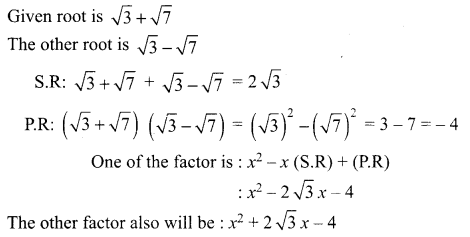The required polynomial equation of minimum degree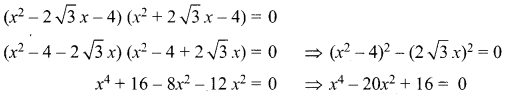Question 3.
If the roots of the equation x3 + px2 + qx + r = 0 are in A.P then show that 2p3 – 9pq + 27 r = 0.
Solution:
Let the roots of the given equation is a – d, a, a + d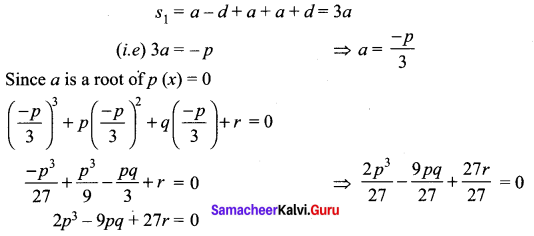Question 4.
Solve 27x3 + 42x2 – 28x -8 = 0 given that its roots are in geometric progressive.
Solution: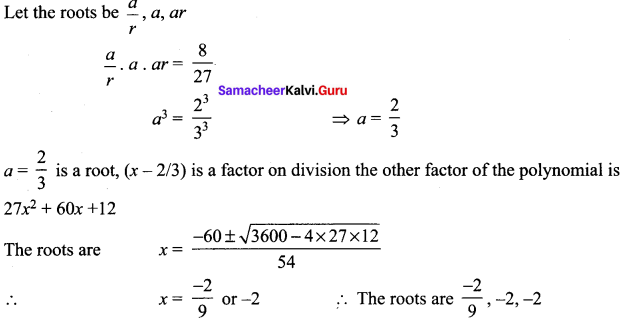Question 5.
Solve the equation 15x3 – 23x2 + 9x – 1 = 0. Where roots are in H.P.
Solution: